# COMPSCI 230 Discrete Mathematics for Computer Science Counting

• Slides: 77COMPSCI 230 Discrete Mathematics for Computer Science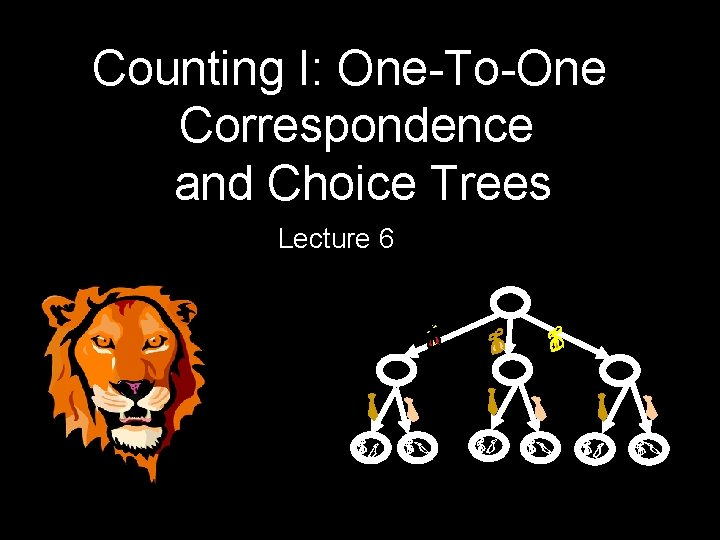Counting I: One-To-One Correspondence and Choice Trees Lecture 6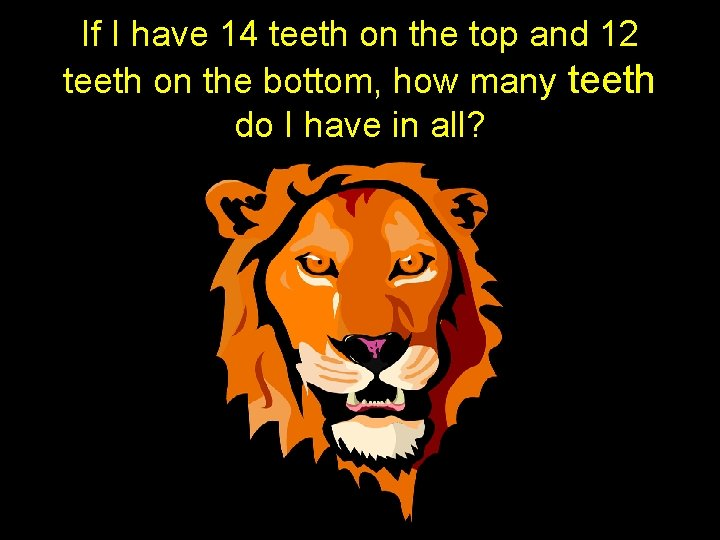If I have 14 teeth on the top and 12 teeth on the bottom, how many teeth do I have in all?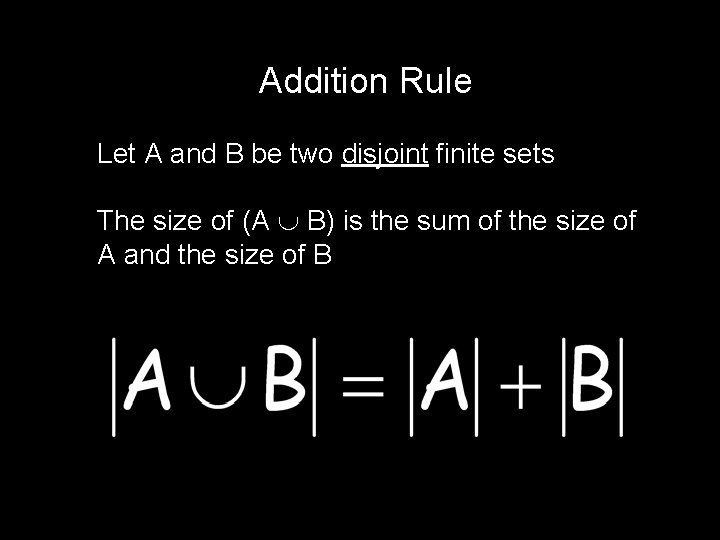Addition Rule Let A and B be two disjoint finite sets The size of (A B) is the sum of the size of A and the size of BAddition Rule (2 possibly overlapping sets) Let A and B be two finite sets |A B| = |A| + |B| - |A B|Addition of multiple disjoint sets: Let A 1, A 2, A 3, …, An be disjoint, finite sets.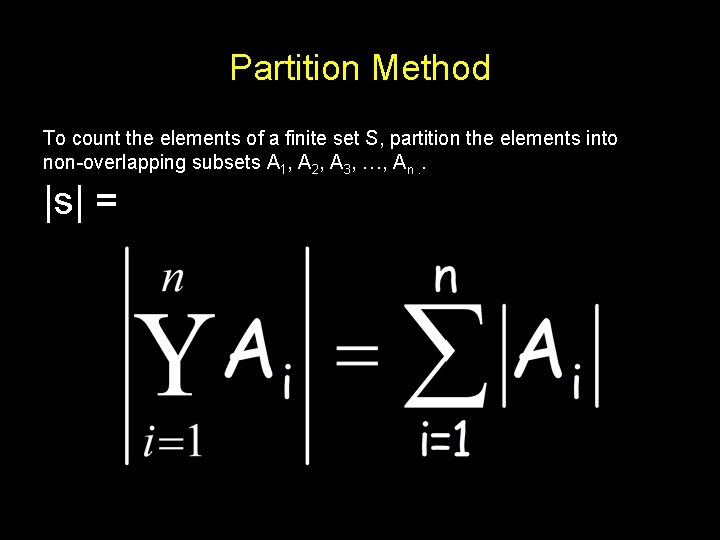Partition Method To count the elements of a finite set S, partition the elements into non-overlapping subsets A 1, A 2, A 3, …, An. . |s| =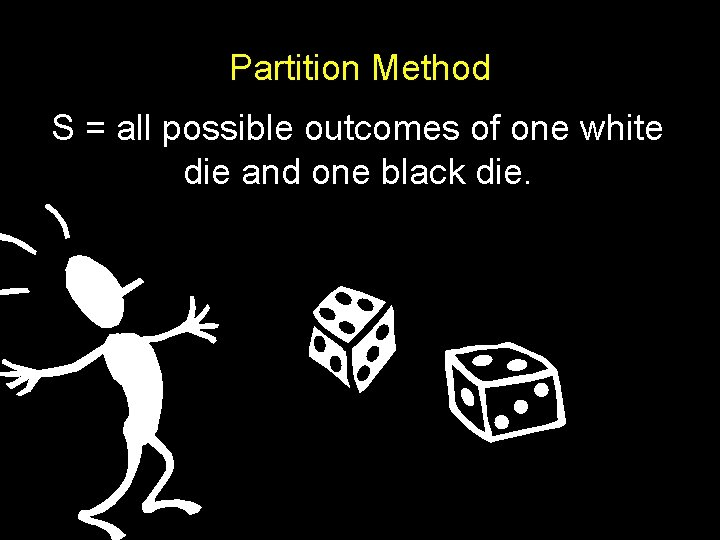Partition Method S = all possible outcomes of one white die and one black die.Partition Method S = all possible outcomes of one white die and one black die. Partition S into 6 sets: A 1 = the set of outcomes where the white die is 1. A 2 = the set of outcomes where the white die is 2. A 3 = the set of outcomes where the white die is 3. A 4 = the set of outcomes where the white die is 4. A 5 = the set of outcomes where the white die is 5. A 6 = the set of outcomes where the white die is 6. Each of 6 disjoint sets have size 6 = 36 outcomesPartition Method S = all possible outcomes where the white die and the black die have different values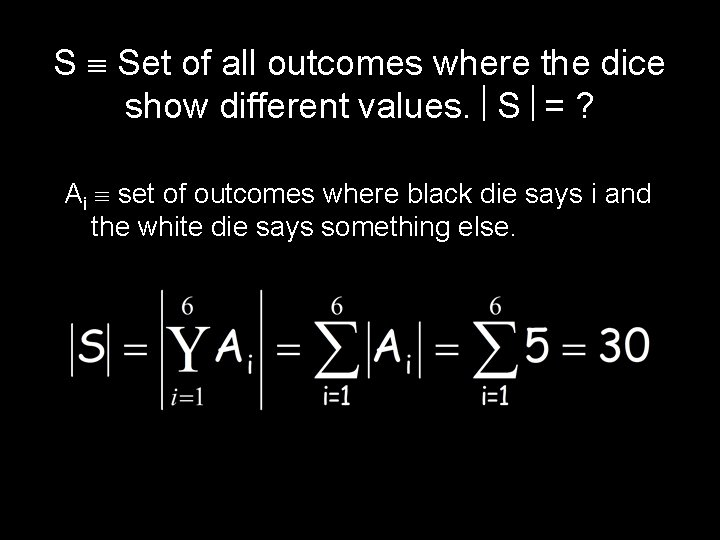S Set of all outcomes where the dice show different values. S = ? Ai set of outcomes where black die says i and the white die says something else.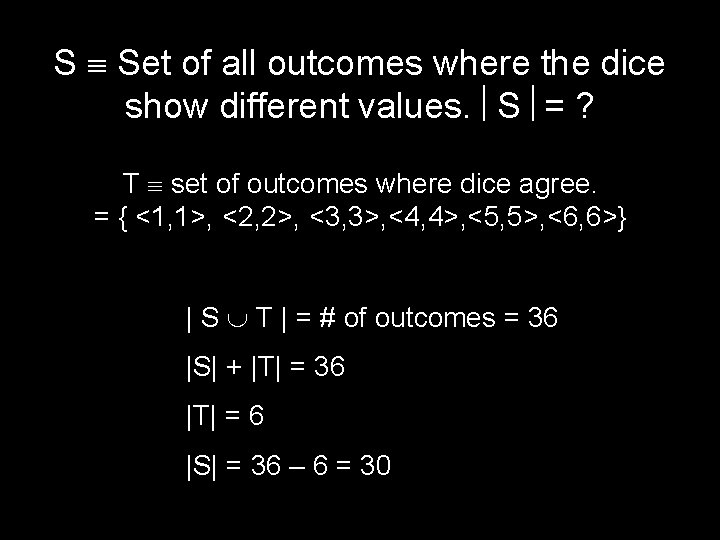S Set of all outcomes where the dice show different values. S = ? T set of outcomes where dice agree. = { <1, 1>, <2, 2>, <3, 3>, <4, 4>, <5, 5>, <6, 6>} | S T | = # of outcomes = 36 |S| + |T| = 36 |T| = 6 |S| = 36 – 6 = 30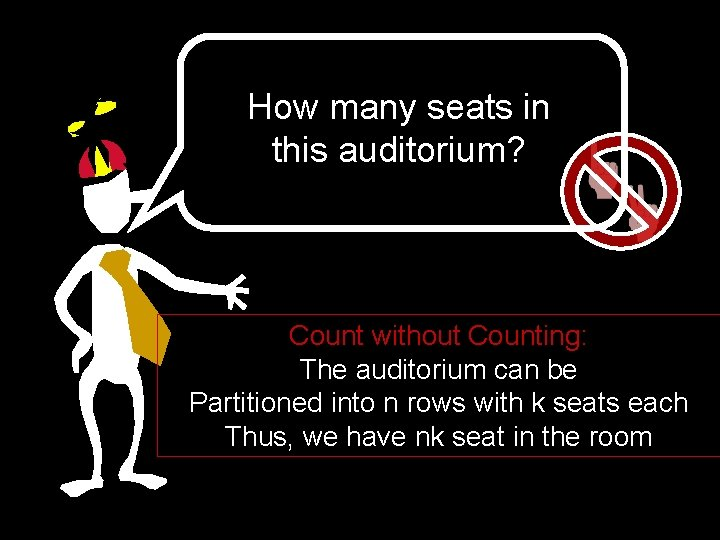How many seats in this auditorium? Count without Counting: The auditorium can be Partitioned into n rows with k seats each Thus, we have nk seat in the room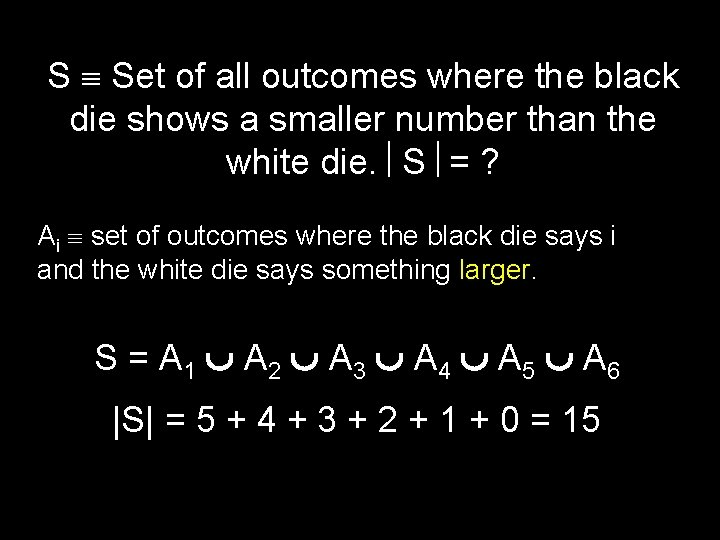S Set of all outcomes where the black die shows a smaller number than the white die. S = ? Ai set of outcomes where the black die says i and the white die says something larger. S = A 1 A 2 A 3 A 4 A 5 A 6 |S| = 5 + 4 + 3 + 2 + 1 + 0 = 15S Set of all outcomes where the black die shows a smaller number than the white die. S = ? L set of all outcomes where the black die shows a larger number than the white die. S + L = 30 It is clear by symmetry that | S | = | L |. Therefore | S | = 15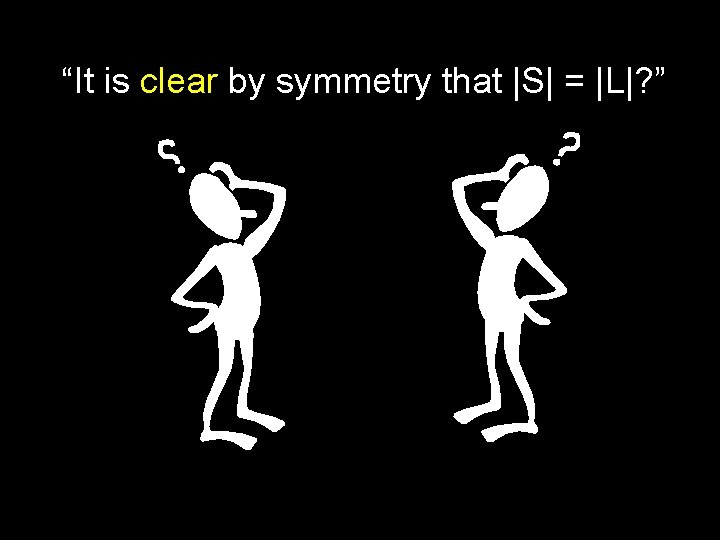“It is clear by symmetry that |S| = |L|? ”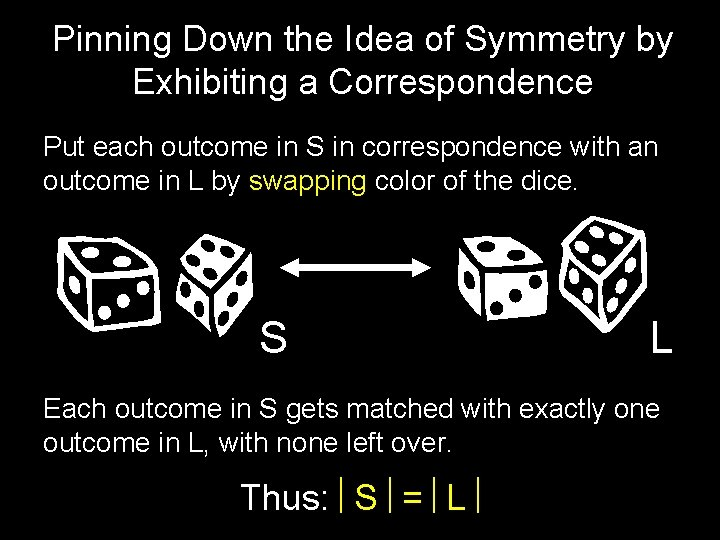Pinning Down the Idea of Symmetry by Exhibiting a Correspondence Put each outcome in S in correspondence with an outcome in L by swapping color of the dice. S L Each outcome in S gets matched with exactly one outcome in L, with none left over. Thus: S = LLet f : A B Be a Function From a Set A to a Set B f is 1 -1 if and only if x, y A, x y f(x) f(y) f is onto if and only if z B x A f(x) = z There Exists For EveryLet’s Restrict Our Attention to Finite Sets A B 1 -1 f : A B | A | ≤ | B |B A onto f : A B | A | ≥ | B |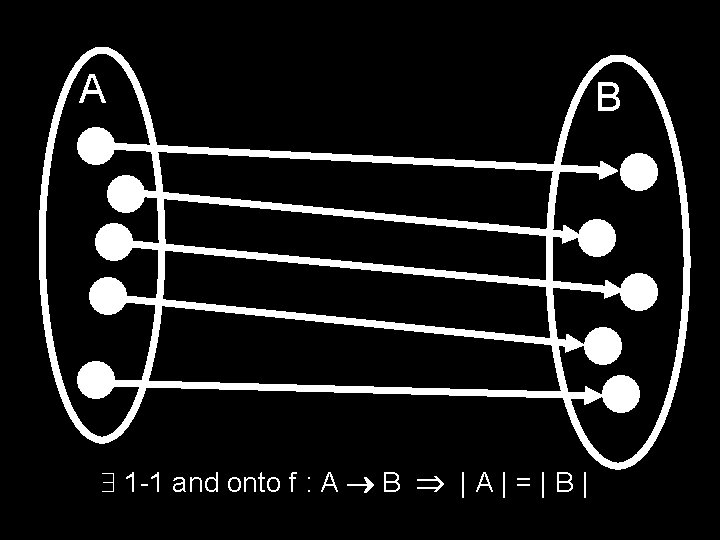A 1 -1 and onto f : A B | A | = | Bf is 1 -1 and onto means f-1 is unique f is a way of pairing up elements A B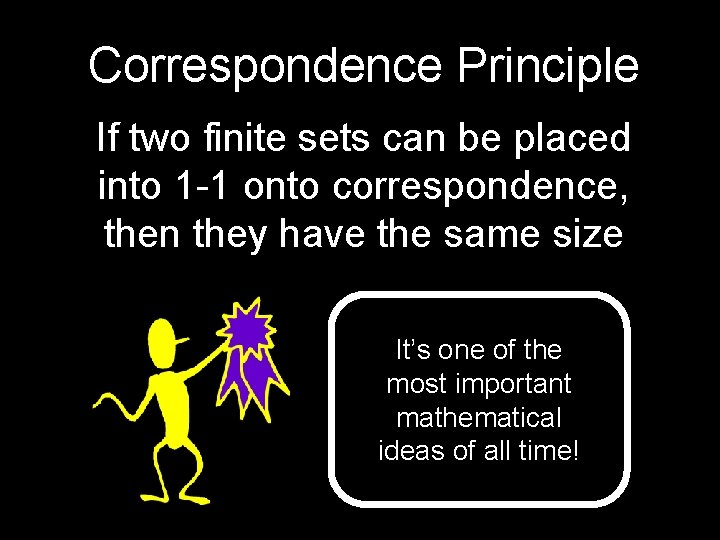Correspondence Principle If two finite sets can be placed into 1 -1 onto correspondence, then they have the same size It’s one of the most important mathematical ideas of all time!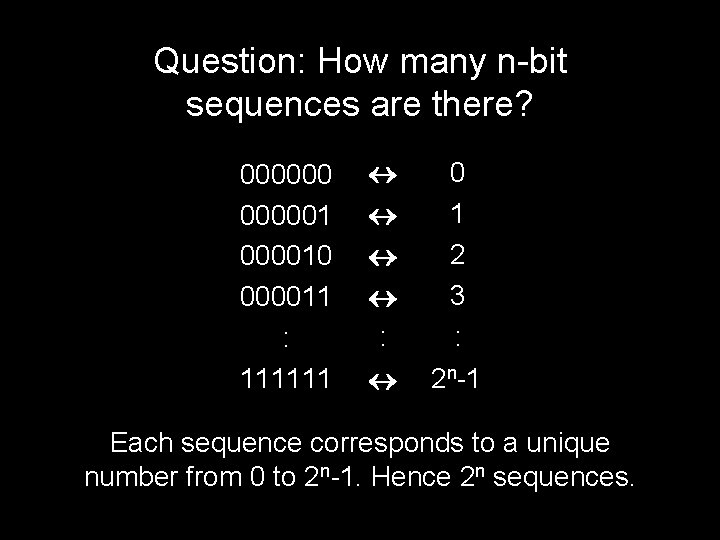Question: How many n-bit sequences are there? 0000001 000010 000011 : 111111 : 0 1 2 3 : 2 n-1 Each sequence corresponds to a unique number from 0 to 2 n-1. Hence 2 n sequences.A = { a, b, c, d, e } Has Many Subsets {a}, {a, b}, {a, d, e}, {a, b, c, d, e}, {e}, Ø, … The entire set and the empty set are subsets with all the rights and privileges pertaining theretoQuestion: How Many Subsets Can Be Made From The Elements of a 5 Element Set? { a b c d e 0 1 1 0 1 b c e } 1 means “TAKE IT” 0 means “LEAVE IT” Each subset corresponds to a 5 -bit sequence (using the “take it or leave it” code)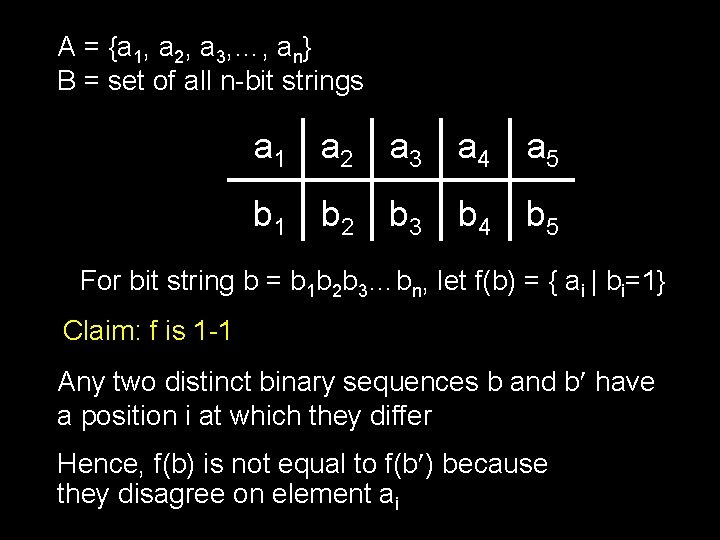A = {a 1, a 2, a 3, …, an} B = set of all n-bit strings a 1 a 2 a 3 a 4 a 5 b 1 b 2 b 3 b 4 b 5 For bit string b = b 1 b 2 b 3…bn, let f(b) = { ai | bi=1} Claim: f is 1 -1 Any two distinct binary sequences b and b have a position i at which they differ Hence, f(b) is not equal to f(b ) because they disagree on element aiA = {a 1, a 2, a 3, …, an} B = set of all n-bit strings a 1 a 2 a 3 a 4 a 5 b 1 b 2 b 3 b 4 b 5 For bit string b = b 1 b 2 b 3…bn, let f(b) = { ai | bi=1} Claim: f is onto Let S be a subset of {a 1, …, an}. Define bk = 1 if ak in S and bk = 0 otherwise. Note that f(b 1 b 2…bn) = S.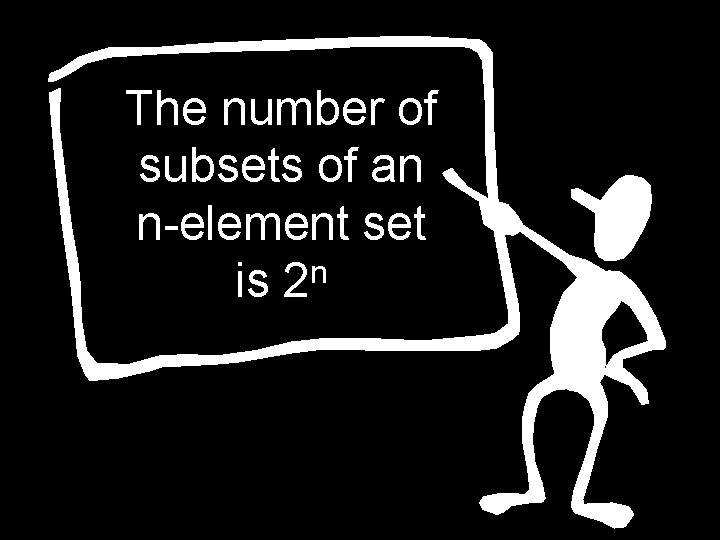The number of subsets of an n-element set n is 2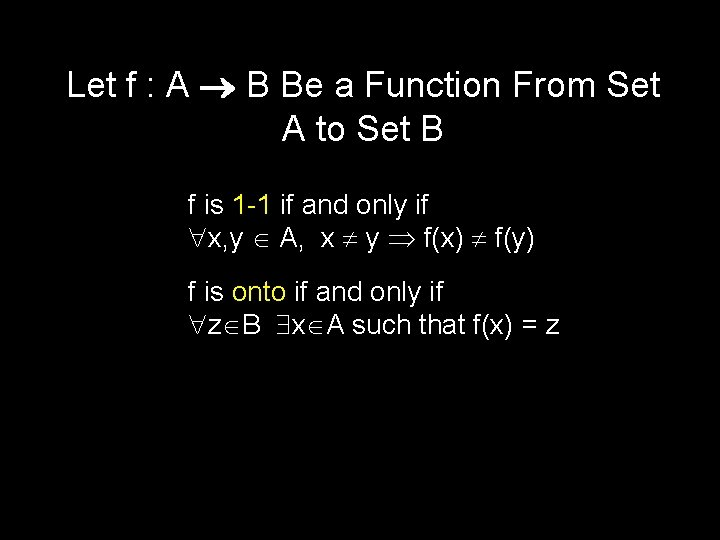Let f : A B Be a Function From Set A to Set B f is 1 -1 if and only if x, y A, x y f(x) f(y) f is onto if and only if z B x A such that f(x) = z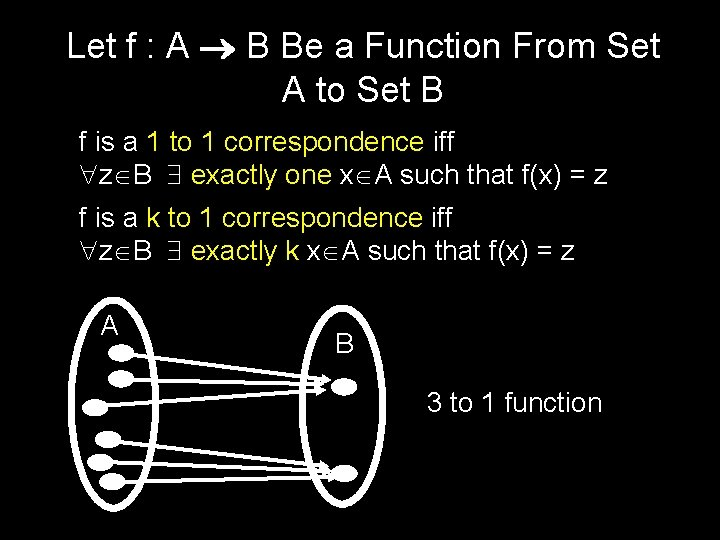Let f : A B Be a Function From Set A to Set B f is a 1 to 1 correspondence iff z B exactly one x A such that f(x) = z f is a k to 1 correspondence iff z B exactly k x A such that f(x) = z A B 3 to 1 functionTo count the number of horses in a barn, we can count the number of hoofs and then divide by 4If a finite set A has a k-to-1 correspondence to finite set B, then |B| = |A|/k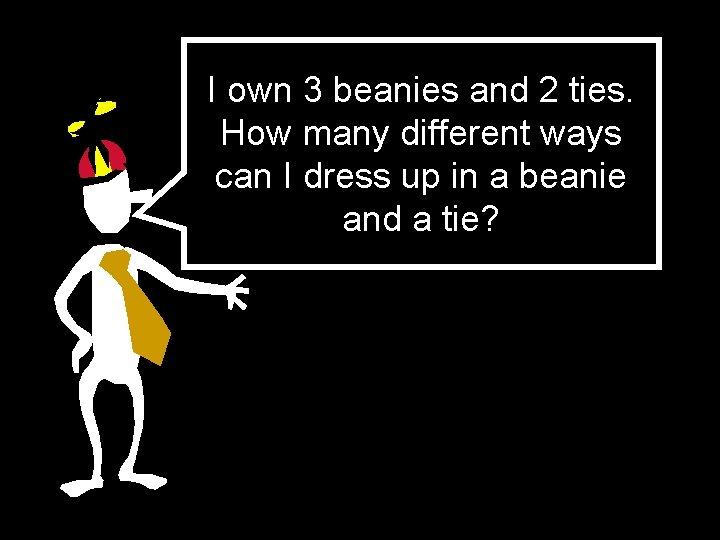I own 3 beanies and 2 ties. How many different ways can I dress up in a beanie and a tie?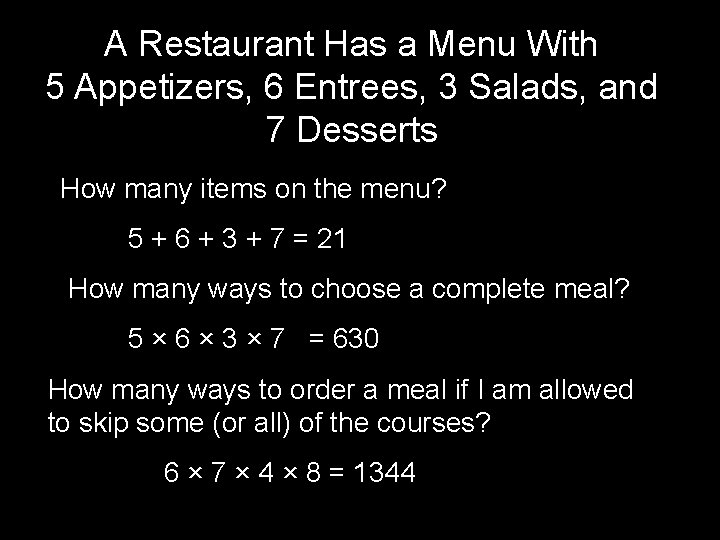A Restaurant Has a Menu With 5 Appetizers, 6 Entrees, 3 Salads, and 7 Desserts How many items on the menu? 5 + 6 + 3 + 7 = 21 How many ways to choose a complete meal? 5 × 6 × 3 × 7 = 630 How many ways to order a meal if I am allowed to skip some (or all) of the courses? 6 × 7 × 4 × 8 = 1344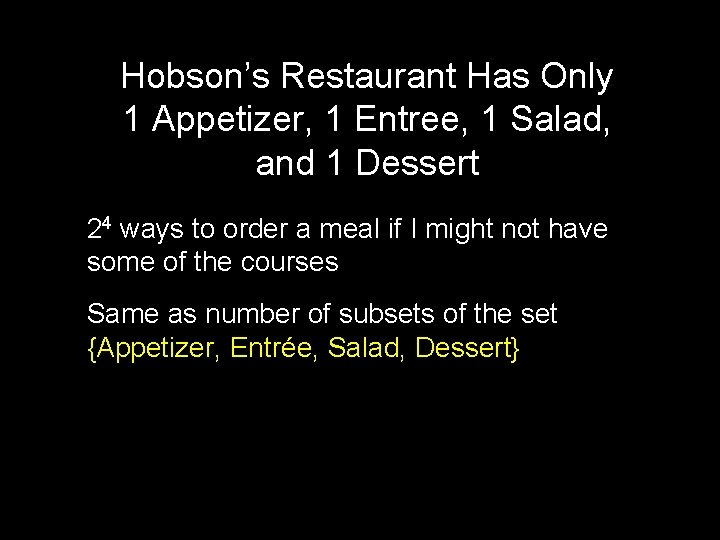Hobson’s Restaurant Has Only 1 Appetizer, 1 Entree, 1 Salad, and 1 Dessert 24 ways to order a meal if I might not have some of the courses Same as number of subsets of the set {Appetizer, Entrée, Salad, Dessert}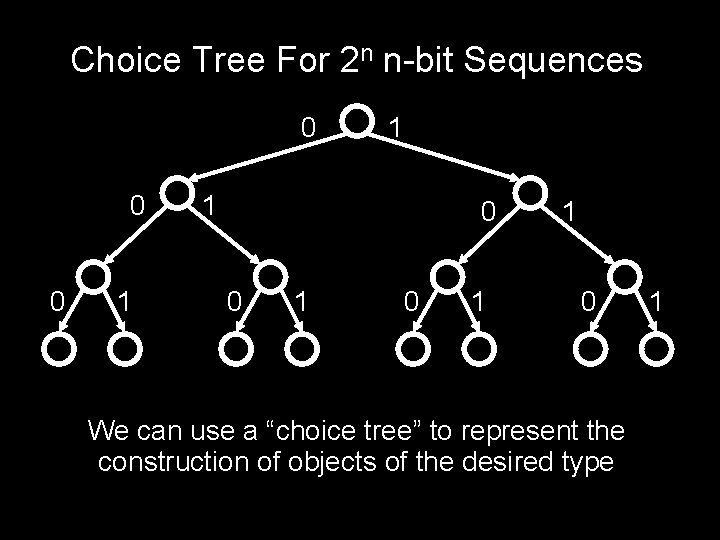Choice Tree For 2 n n-bit Sequences 0 0 0 1 1 1 0 0 1 1 0 We can use a “choice tree” to represent the construction of objects of the desired type 1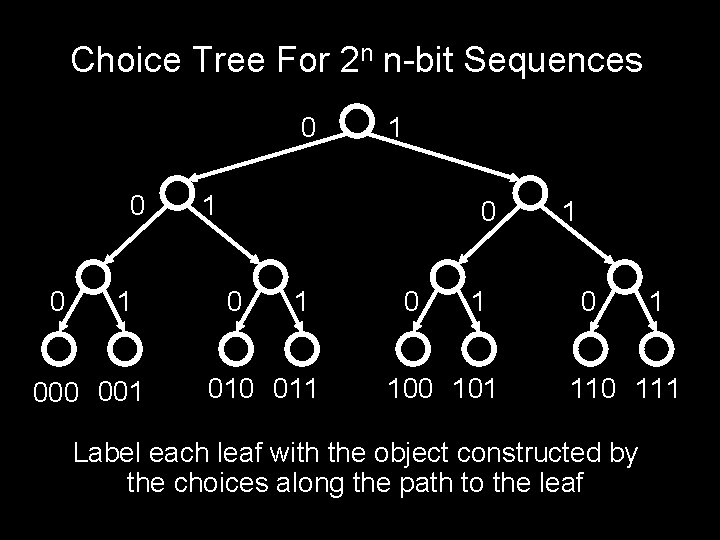Choice Tree For 2 n n-bit Sequences 0 0 0 1 000 001 1 1 0 0 1 010 011 0 1 100 101 1 0 1 110 111 Label each leaf with the object constructed by the choices along the path to the leaf0 0 0 1 1 1 0 0 1 2 choices for first bit × 2 choices for second bit × 2 choices for third bit : : × 2 choices for the nth 1 0 1Leaf Counting Lemma Let T be a depth-n tree when each node at depth 0 i n-1 has Pi+1 children The number of leaves of T is given by: P 1 P 2…PnChoice Tree A choice tree is a rooted, directed tree with an object called a “choice” associated with each edge and a label on each leafA choice tree provides a “choice tree representation” of a set S, if 1. Each leaf label is in S, and each element of S is some leaf label 2. No two leaf labels are the same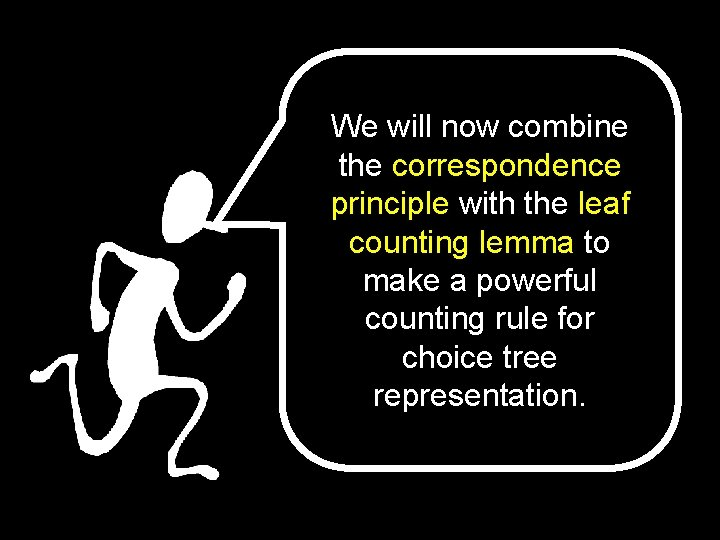We will now combine the correspondence principle with the leaf counting lemma to make a powerful counting rule for choice tree representation.Product Rule IF set S has a choice tree representation with P 1 possibilities for the first choice, P 2 for the second, P 3 for the third, and so on, THEN there are P 1 P 2 P 3…Pn objects in S Proof: There are P 1 P 2 P 3…Pn leaves of the choice tree which are in 1 -1 onto correspondence with the elements of S.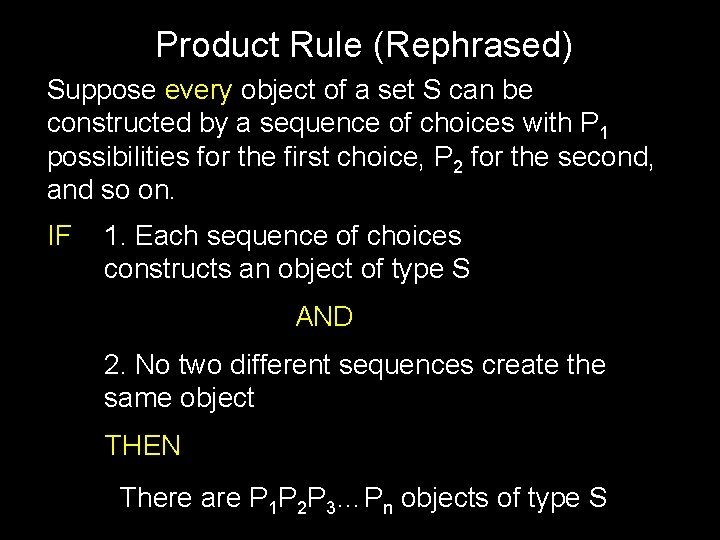Product Rule (Rephrased) Suppose every object of a set S can be constructed by a sequence of choices with P 1 possibilities for the first choice, P 2 for the second, and so on. IF 1. Each sequence of choices constructs an object of type S AND 2. No two different sequences create the same object THEN There are P 1 P 2 P 3…Pn objects of type S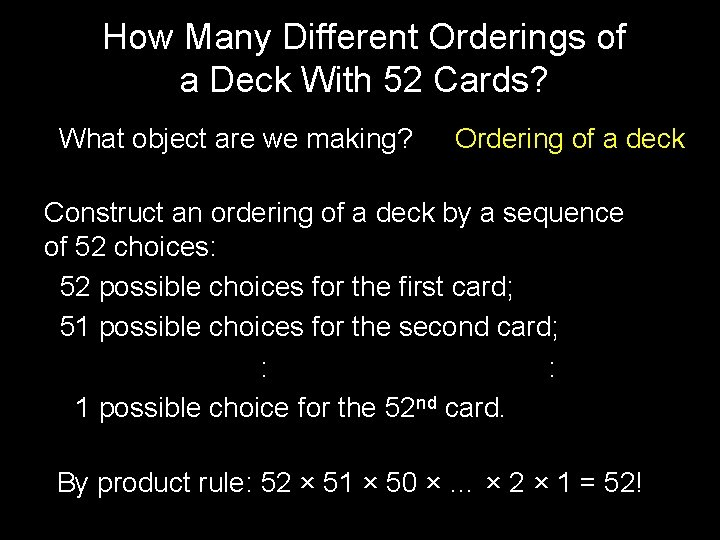How Many Different Orderings of a Deck With 52 Cards? What object are we making? Ordering of a deck Construct an ordering of a deck by a sequence of 52 choices: 52 possible choices for the first card; 51 possible choices for the second card; : : 1 possible choice for the 52 nd card. By product rule: 52 × 51 × 50 × … × 2 × 1 = 52!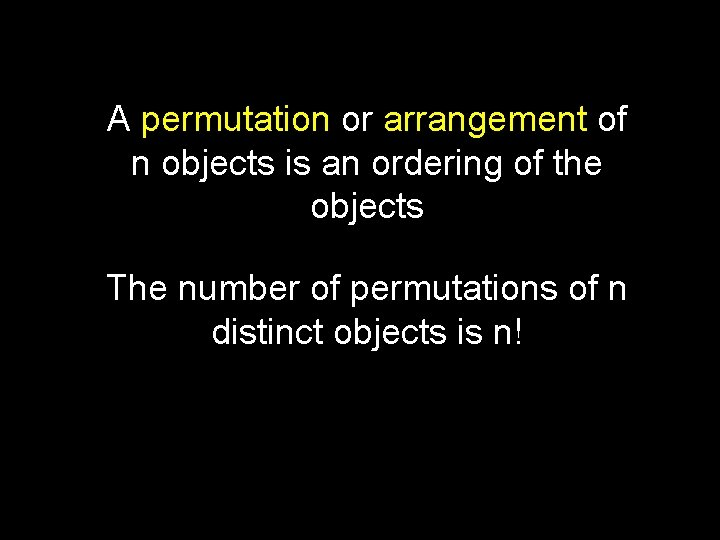A permutation or arrangement of n objects is an ordering of the objects The number of permutations of n distinct objects is n!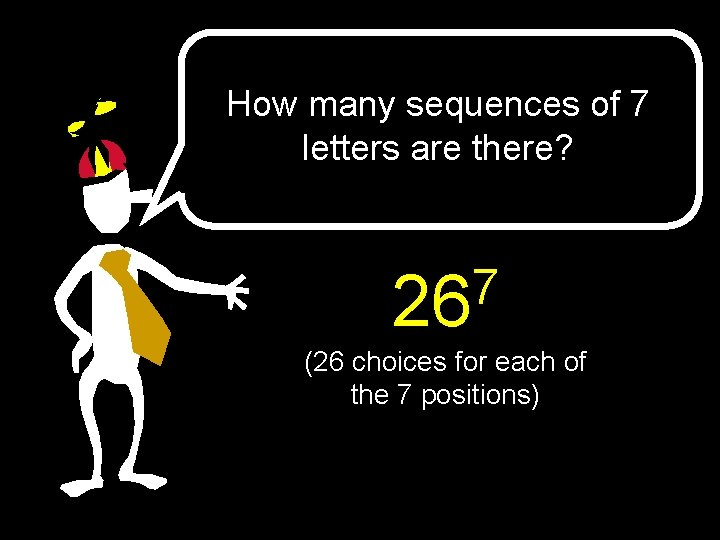How many sequences of 7 letters are there? 7 26 (26 choices for each of the 7 positions)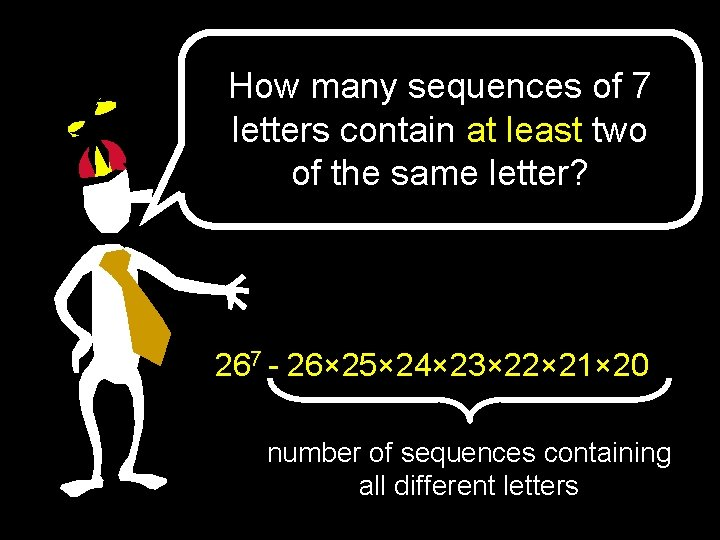How many sequences of 7 letters contain at least two of the same letter? 267 - 26× 25× 24× 23× 22× 21× 20 number of sequences containing all different letters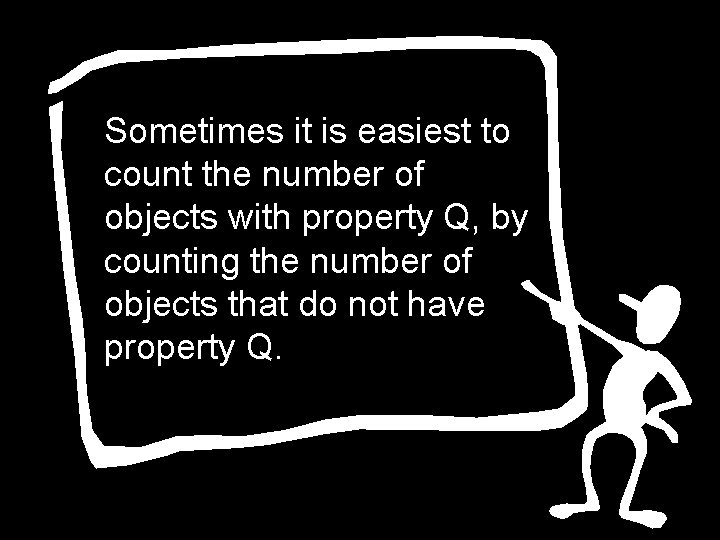Sometimes it is easiest to count the number of objects with property Q, by counting the number of objects that do not have property Q.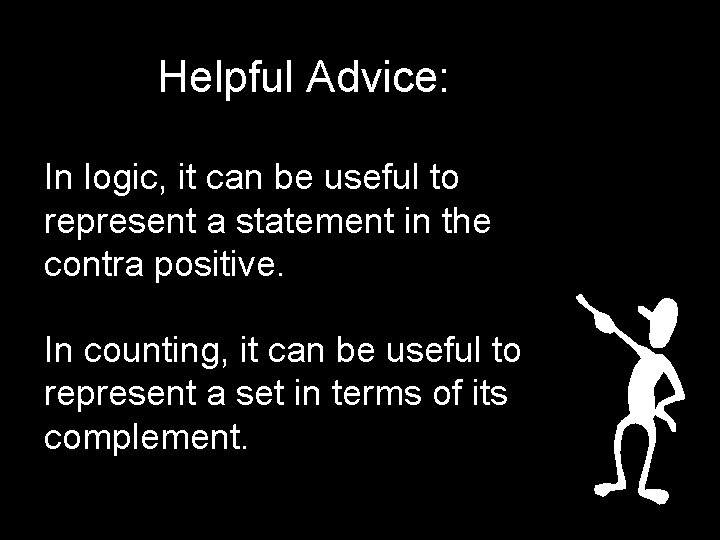Helpful Advice: In logic, it can be useful to represent a statement in the contra positive. In counting, it can be useful to represent a set in terms of its complement.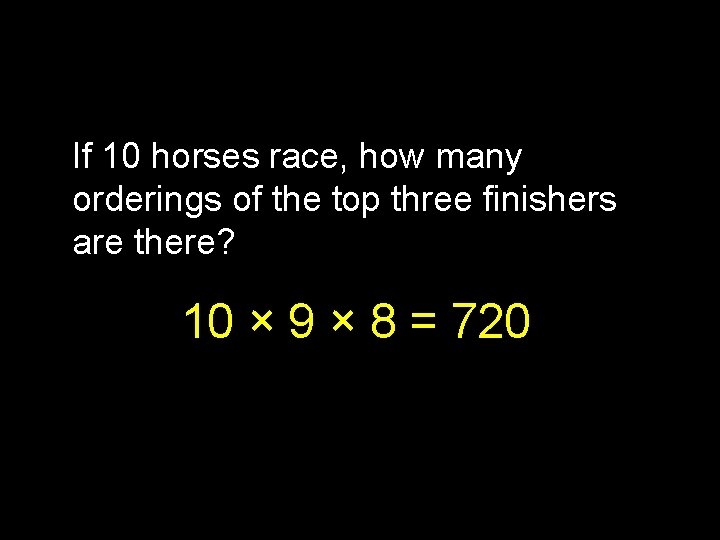If 10 horses race, how many orderings of the top three finishers are there? 10 × 9 × 8 = 720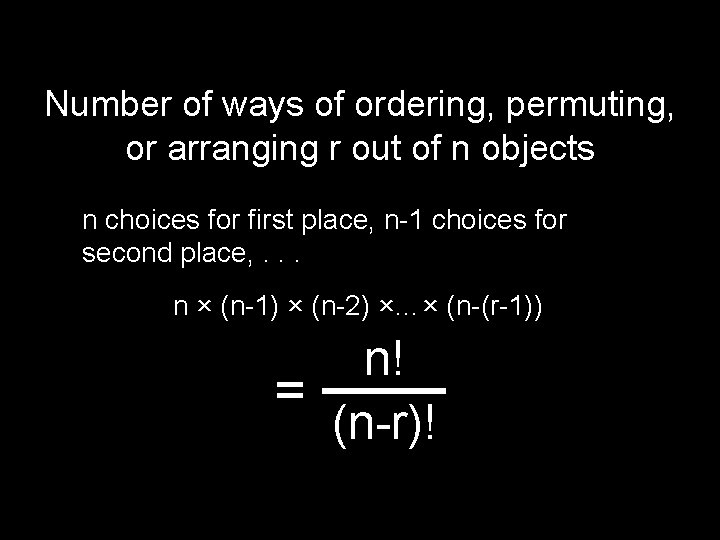Number of ways of ordering, permuting, or arranging r out of n objects n choices for first place, n-1 choices for second place, . . . n × (n-1) × (n-2) ×…× (n-(r-1)) n! = (n-r)!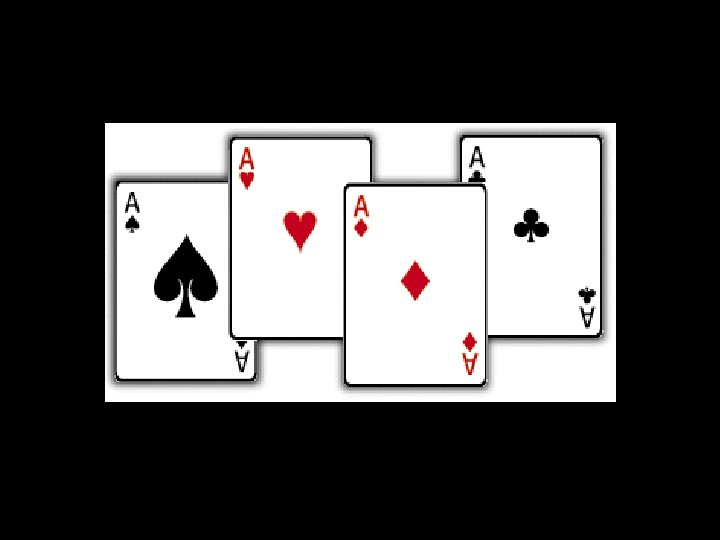Ordered Versus Unordered From a deck of 52 cards how many ordered pairs can be formed? 52 × 51 How many unordered pairs? 52× 51 / 2 divide by overcount Each unordered pair is listed twice on a list of the ordered pairsOrdered Versus Unordered From a deck of 52 cards how many ordered pairs can be formed? 52 × 51 How many unordered pairs? 52× 51 / 2 divide by overcount We have a 2 -1 map from ordered pairs to unordered pairs. Hence #unordered pairs = (#ordered pairs)/2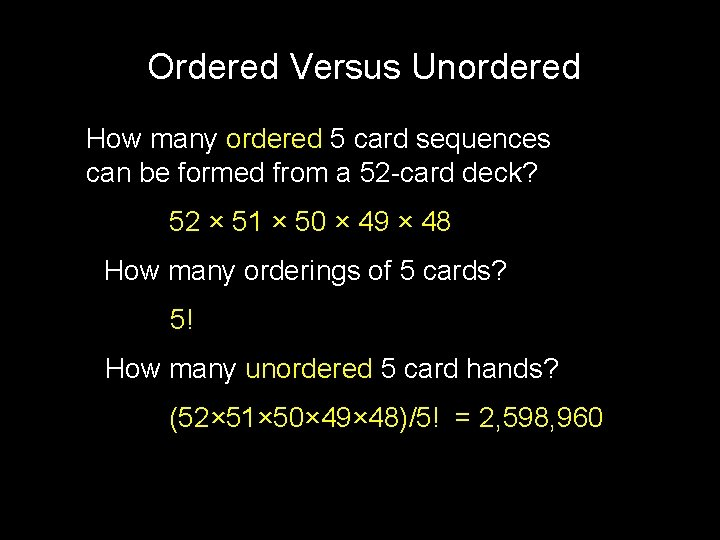Ordered Versus Unordered How many ordered 5 card sequences can be formed from a 52 -card deck? 52 × 51 × 50 × 49 × 48 How many orderings of 5 cards? 5! How many unordered 5 card hands? (52× 51× 50× 49× 48)/5! = 2, 598, 960A combination or choice of r out of n objects is an (unordered) set of r of the n objects The number of r combinations of n objects: n! n = r!(n-r)! r n “choose” rThe number of subsets of size r that can be formed from an n-element set is: n! n = r!(n-r)! rProduct Rule (Rephrased) Suppose every object of a set S can be constructed by a sequence of choices with P 1 possibilities for the first choice, P 2 for the second, and so on. IF 1. Each sequence of choices constructs an object of type S AND 2. No two different sequences create the same object THEN There are P 1 P 2 P 3…Pn objects of type S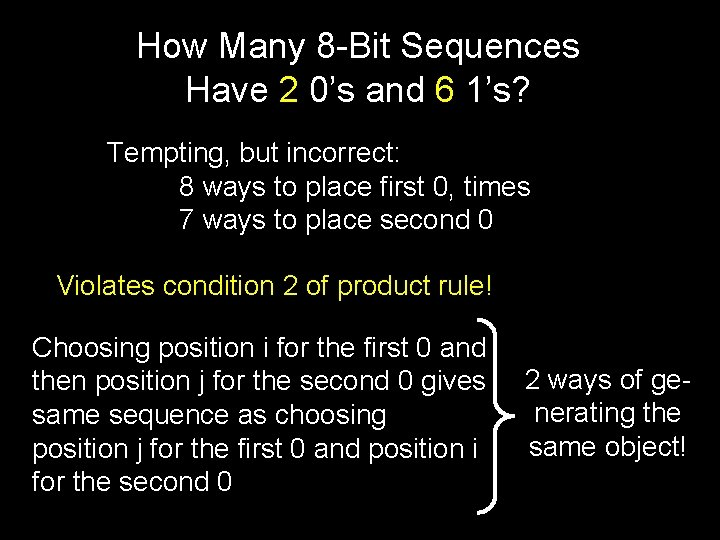How Many 8 -Bit Sequences Have 2 0’s and 6 1’s? Tempting, but incorrect: 8 ways to place first 0, times 7 ways to place second 0 Violates condition 2 of product rule! Choosing position i for the first 0 and then position j for the second 0 gives same sequence as choosing position j for the first 0 and position i for the second 0 2 ways of generating the same object!How Many 8 -Bit Sequences Have 2 0’s and 6 1’s? 1. Choose the set of 2 positions to put the 0’s. The 1’s are forced. 8 2 2. Choose the set of 6 positions to put the 1’s. The 0’s are forced. 8 6Symmetry In The Formula n! n n = = r r!(n-r)! n-r “# of ways to pick r out of n elements” = “# of ways to choose the (n-r) elements to omit”How Many Hands Have at Least 3 As? 4 3 = 4 ways of picking 3 out of 4 aces 49 2 = 1176 ways of picking 2 cards out of the remaining 49 cards 4 × 1176 = 4704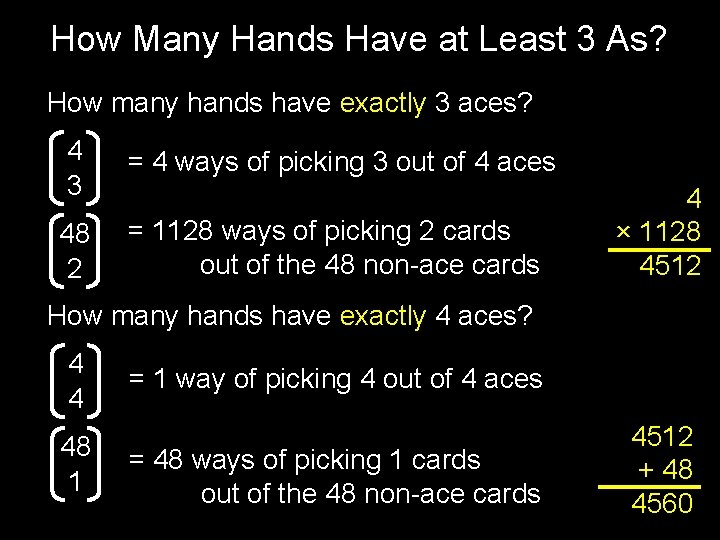How Many Hands Have at Least 3 As? How many hands have exactly 3 aces? 4 3 = 4 ways of picking 3 out of 4 aces 48 2 = 1128 ways of picking 2 cards out of the 48 non-ace cards 4 × 1128 4512 How many hands have exactly 4 aces? 4 4 48 1 = 1 way of picking 4 out of 4 aces = 48 ways of picking 1 cards out of the 48 non-ace cards 4512 + 48 45604704 4560 At least one of the two counting arguments is not correct!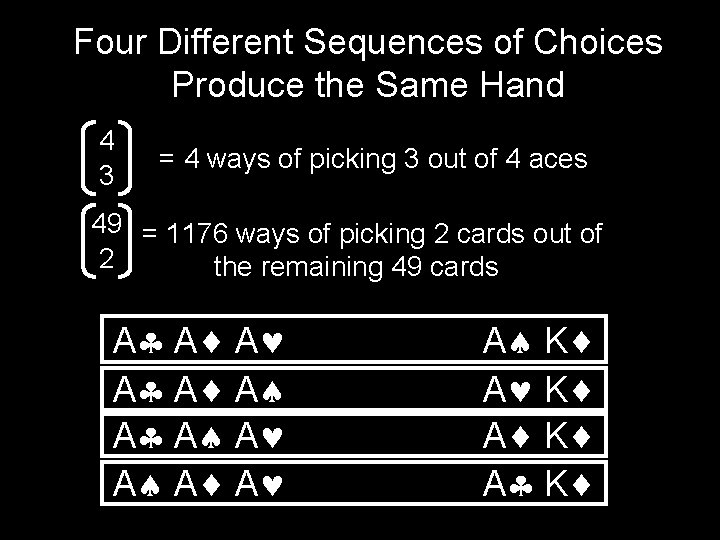Four Different Sequences of Choices Produce the Same Hand 4 3 = 4 ways of picking 3 out of 4 aces 49 = 1176 ways of picking 2 cards out of 2 the remaining 49 cards A A A A K A KIs the other argument correct? How do I avoid fallacious reasoning?REVERSIBILTY CHECK: For each object can I reverse engineer the unique sequence of choices that constructed it?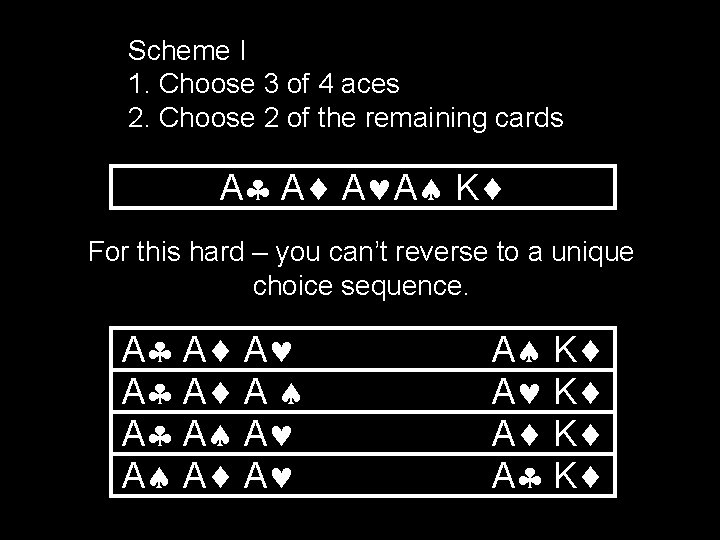Scheme I 1. Choose 3 of 4 aces 2. Choose 2 of the remaining cards A A K For this hard – you can’t reverse to a unique choice sequence. A A A A K A K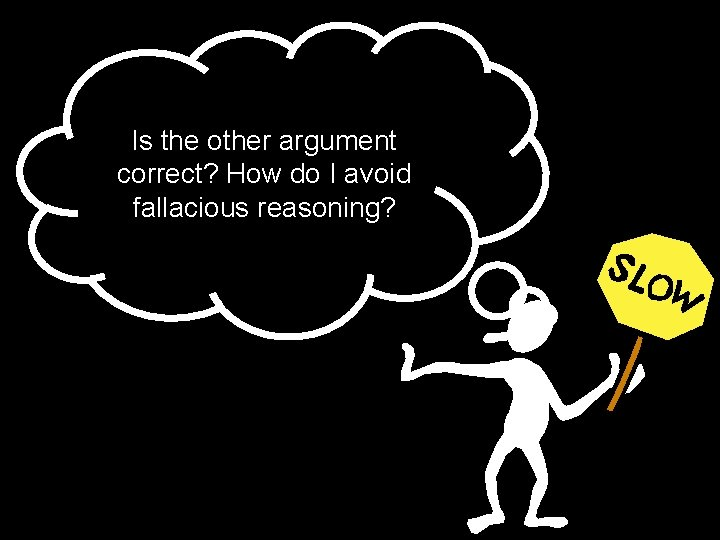Is the other argument correct? How do I avoid fallacious reasoning?Scheme II 1. Choose 3 out of 4 aces 2. Choose 2 out of 48 non-ace cards A A Q A K REVERSE TEST: Aces came from choices in (1) and others came from choices in (2)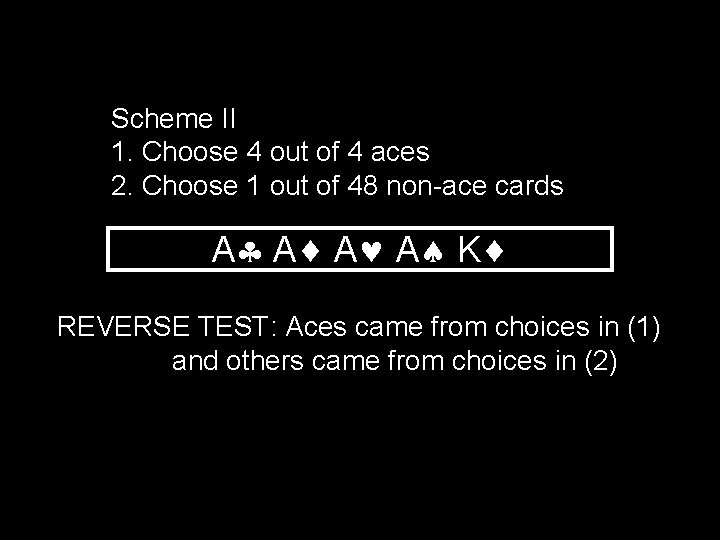Scheme II 1. Choose 4 out of 4 aces 2. Choose 1 out of 48 non-ace cards A A K REVERSE TEST: Aces came from choices in (1) and others came from choices in (2)Product Rule (Rephrased) Suppose every object of a set S can be constructed by a sequence of choices with P 1 possibilities for the first choice, P 2 for the second, and so on. IF 1. Each sequence of choices constructs an object of type S AND 2. No two different sequences create the same object THEN There are P 1 P 2 P 3…Pn objects of type S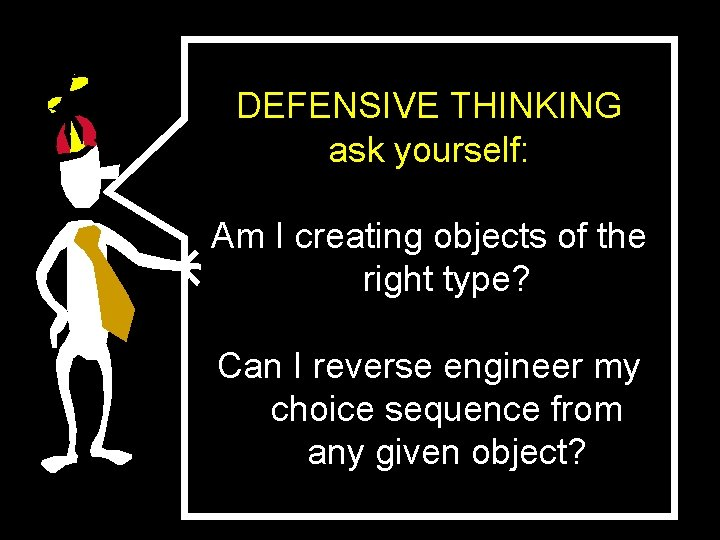DEFENSIVE THINKING ask yourself: Am I creating objects of the right type? Can I reverse engineer my choice sequence from any given object?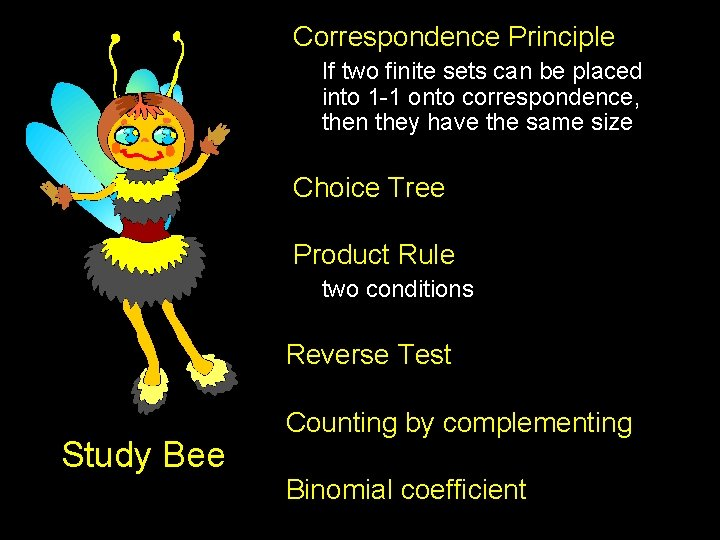Correspondence Principle If two finite sets can be placed into 1 -1 onto correspondence, then they have the same size Choice Tree Product Rule two conditions Reverse Test Study Bee Counting by complementing Binomial coefficient